## Описание презентации Physics of Semiconductor Devices Lecture 6. по слайдам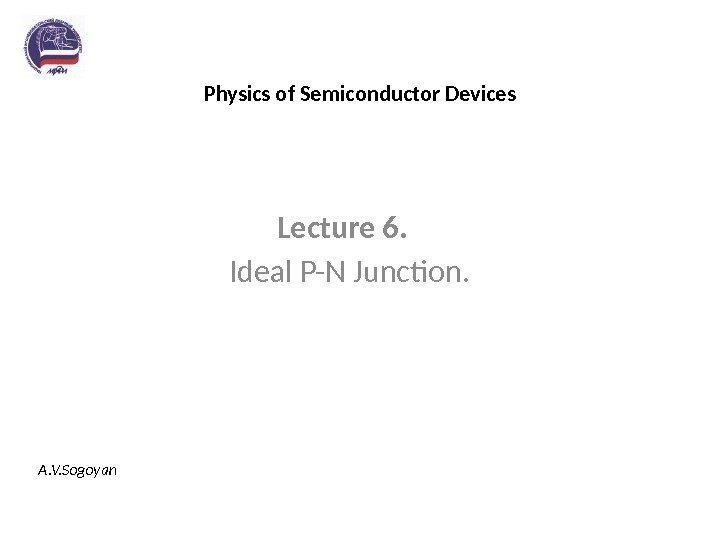Physics of Semiconductor Devices Lecture 6. Ideal P-N Junction. A. V. Sogoyan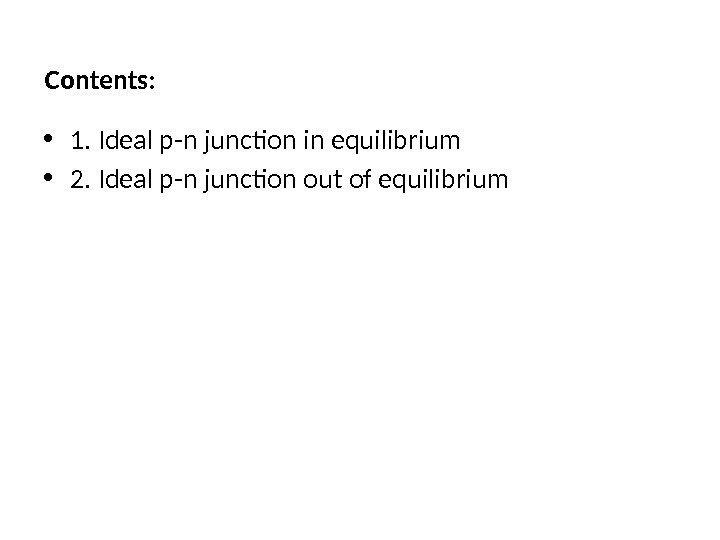Contents: • 1. Ideal p-n junction in equilibrium • 2. Ideal p-n junction out of equilibrium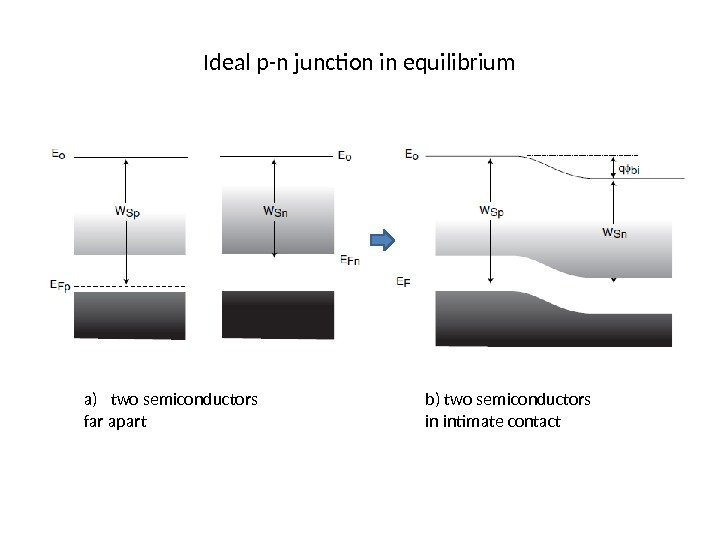Ideal p-n junction in equilibrium a) two semiconductors far apart b) two semiconductors in intimate contact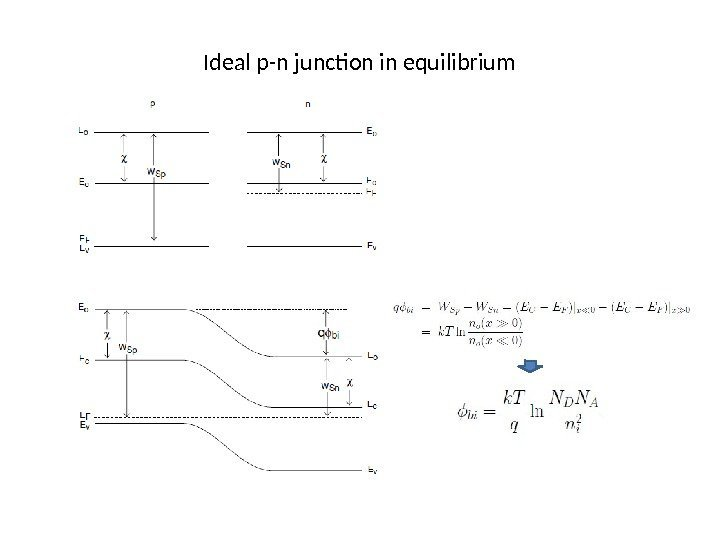Ideal p-n junction in equilibrium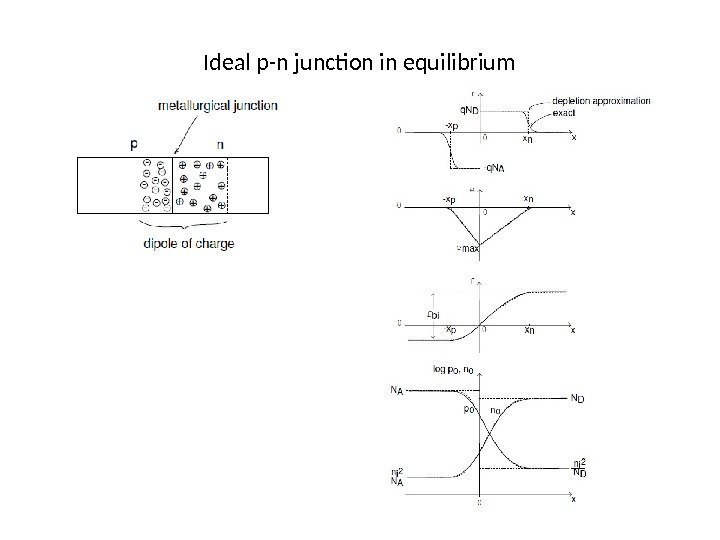Ideal p-n junction in equilibrium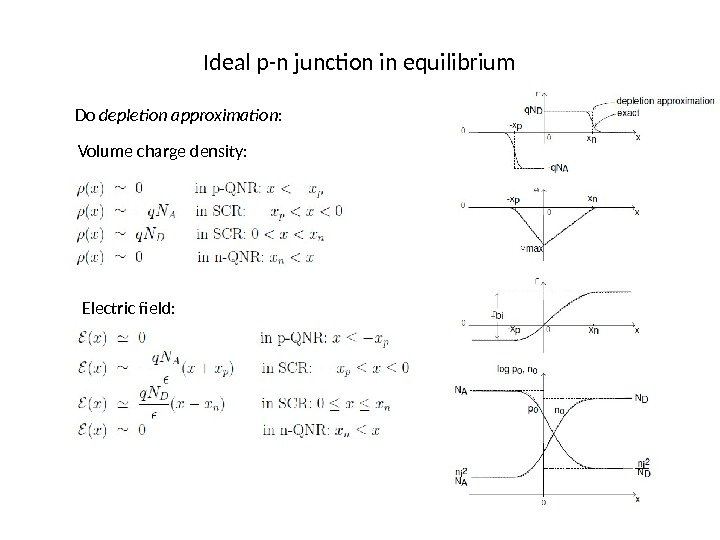Ideal p-n junction in equilibrium Do depletion approximation : Volume charge density: Electric field: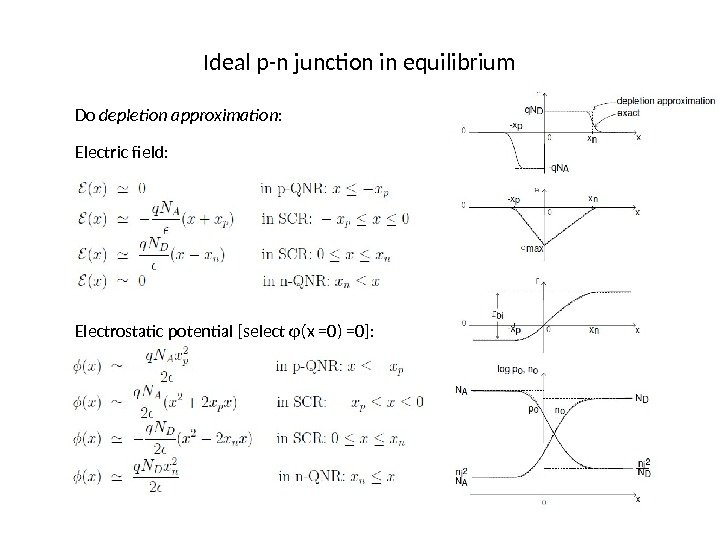Ideal p-n junction in equilibrium Do depletion approximation : Electric field: Electrostatic potential [select φ(x =0) =0]: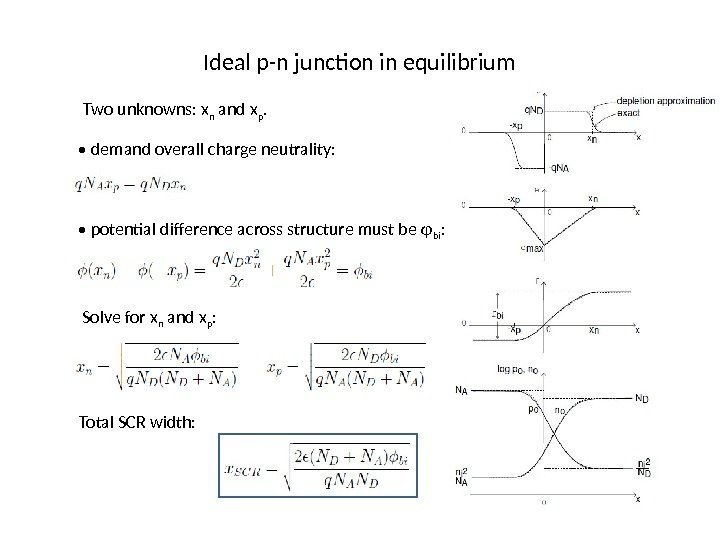Ideal p-n junction in equilibrium Two unknowns: x n and x p. • demand overall charge neutrality: • potential difference across structure must be φ bi : Solve for x n and x p : Total SCR width: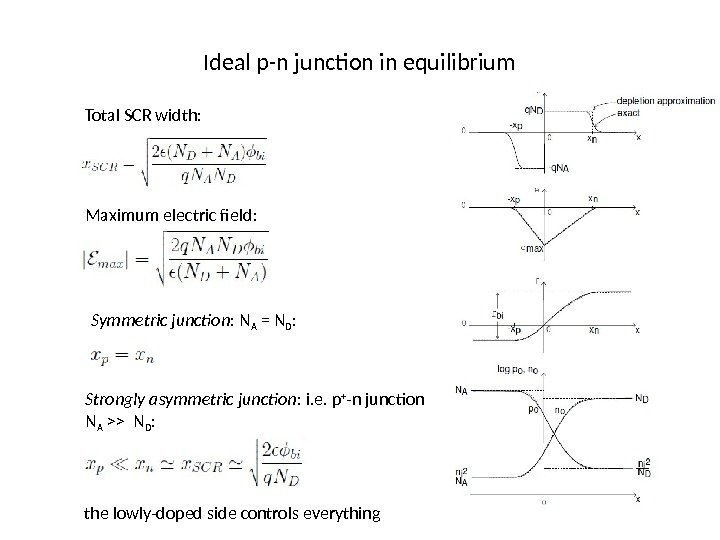Ideal p-n junction in equilibrium Total SCR width: Maximum electric field: Symmetric junction : N A = N D : Strongly asymmetric junction : i. e. p + -n junction N A >> N D : the lowly-doped side controls everything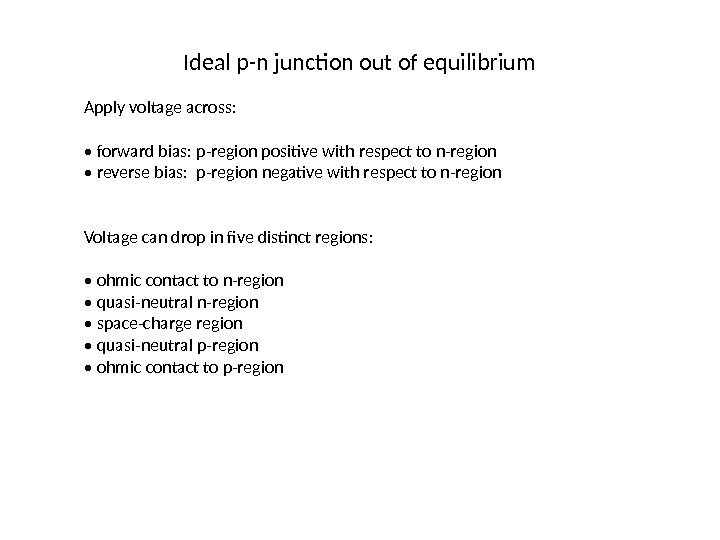Ideal p-n junction out of equilibrium Apply voltage across: • forward bias: p-region positive with respect to n-region • reverse bias: p-region negative with respect to n-region Voltage can drop in five distinct regions: • ohmic contact to n-region • quasi-neutral n-region • space-charge region • quasi-neutral p-region • ohmic contact to p-region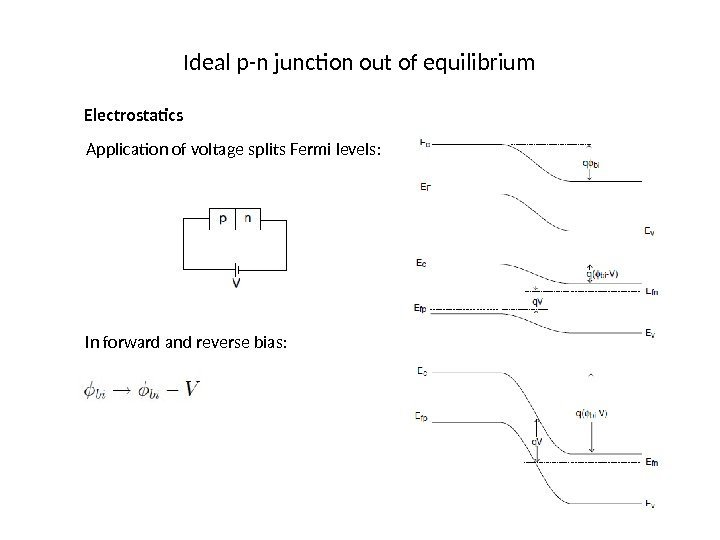Ideal p-n junction out of equilibrium Electrostatics Application of voltage splits Fermi levels: In forward and reverse bias: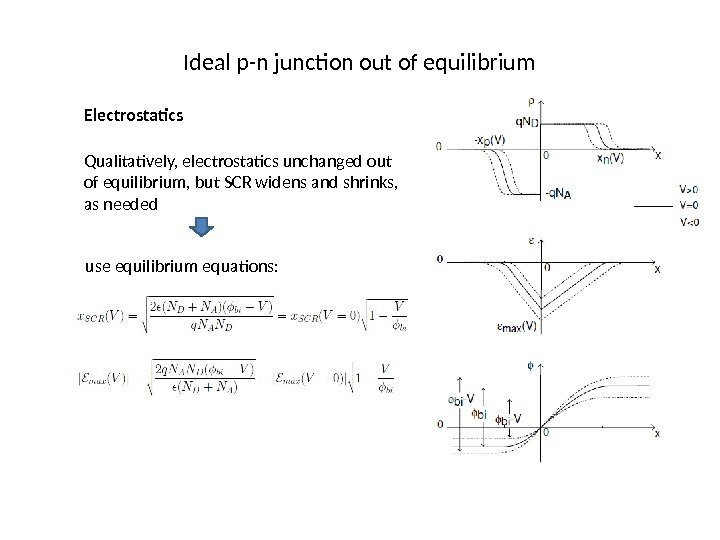Ideal p-n junction out of equilibrium Electrostatics Qualitatively, electrostatics unchanged out of equilibrium, but SCR widens and shrinks, as needed use equilibrium equations: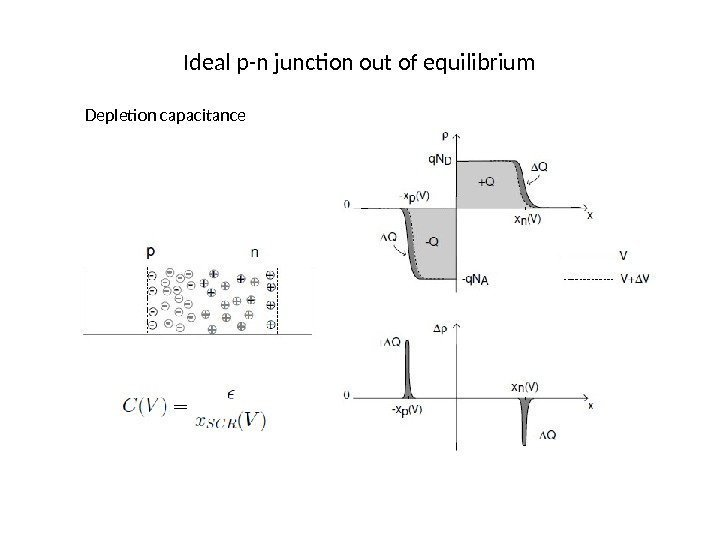Ideal p-n junction out of equilibrium Depletion capacitance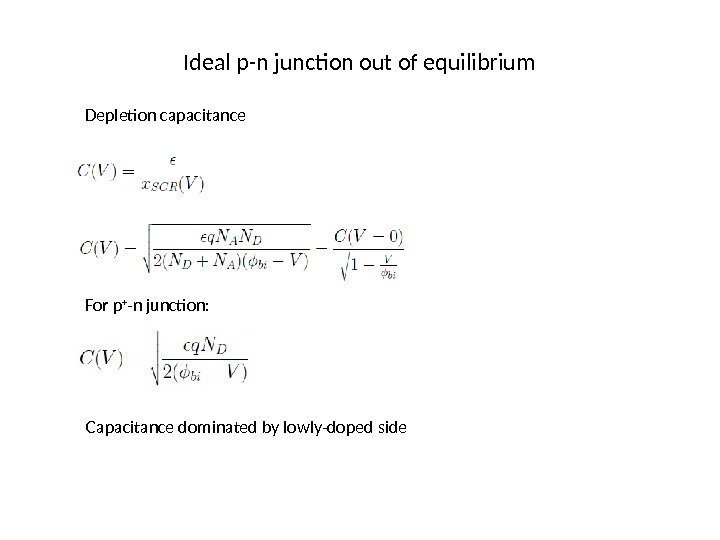Ideal p-n junction out of equilibrium Depletion capacitance For p + -n junction: Capacitance dominated by lowly-doped side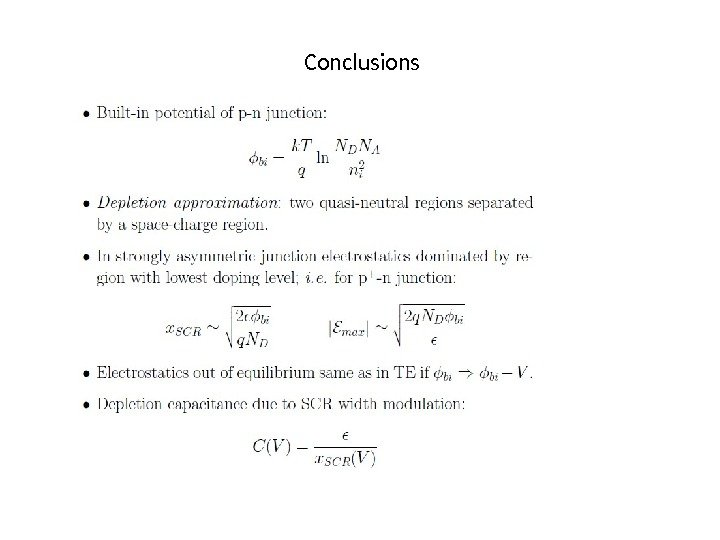Conclusions